# Ligatures for Logic

A ligature in typesetting is a way of presenting two (or more) consecutive characters differently the individual characters would be displayed. For example, “fi” is often rendered with the top of the ‘f’ dotting the ‘i’. Here’s an example from Computer Modern, the default font in LaTeX.Usually the difference is subtle—ordinarily readers are not consciously aware of them—but a ligature could look entirely different from its components. The previous post is an example of the latter: the two-letter abbreviation for a country is rendered as the flag of that country.

I’ve been playing around with Fira Code, a font with ligatures for programming. Fonts like this aim to do for programming what ordinary ligatures do for prose. For example, a programming font might include a ligature to render >= as ≥.

Programming fonts are obviously intended for use in programming, but I personally don’t like the idea of using ligatures in programming. They compromise the simplicity of plain text . They’re supported in some environments but not in others, or they require some fiddly configuration before they’ll work, etc.

Still, I like the aesthetics of Fira Code, particularly in the way it handles logic symbols. Here are some examples comparing a common monospace font and Fira Code.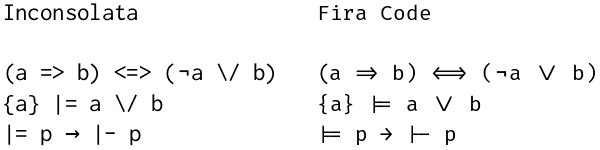The image above is a screen shot of a document created in LibreOffice Writer. The ligatures didn’t work when I tried using them in Microsoft Word.

The Fira Code was designed as a monospace font, but has been extended to include proportional fonts. Fira Code with a proportional font might be useful in prose documents. You could insert a few symbols with a couple key strokes rather than searching for the symbol or entering Unicode.

However, it seems most of Fira Code’s ligatures are only available in monospaced versions of the font. If you use Fira Code in a prose document, you could switch from proportional font to monospace font just for an occasional symbol. It’s unclear whether that would be more or less work than other alternatives.

There’s one place where I believe Fira Code would be ideal: code examples inside a prose document. In that context you care about aesthetics and you want a monospaced font. Here again are some examples comparing Inconsolata and Fira Code.If you use Fira Code font, your code doesn’t change a bit. You can have some aesthetic improvements along with the advantages of working in plain text. But it may not just work without some research and experimentation.

# Modal logic posts## Blog posts about modal logic

I created the image above by asking DALL-E 2 for an image of diamonds and boxes, a sort of pun on the ◇ (“diamond”) and □ (“box”) operators in modal logic.

# Temporal and polymodal logics

My posts on modal logic have mostly been about monomodal logic, logic with one modal operator. This may not seem accurate because I’ve talked about □ (“box”) and ◇ (“diamond”). But these are really just one mode: you can define either in terms of the other.

p = ¬ □ ¬p
p = ¬ ◇ ¬p

## Temporal logic

Temporal logic is an example of a polymodal operator. There are many varieties of temporal logic, but one version defined by Arthur Prior in the 1950s has two basic modes: P (past) and F (future). Prior called his system tense logic but the term temporal logic is more common now.

We can interpret Fp to mean that at some point in the future, p will be the case.

We can interpret Pp to mean at some point in the past p was the case.

In temporal logic we have two analogs of the box operator, and each has its dual counterpart analogous to diamond.

Gp = ¬ F ¬p
Hp = ¬ P ¬p

So Gp can be read as “from now on p will be the case.”

And Hp can be read as “always up until now p has been the case.”

The combination GFp can be interpreted as “eventually p.”

The combination PHp can be interpreted as “up until some point in the past, p.”

I’ve mentioned before how Kripke semantics—models of “accessible worlds”—sounds like science fiction. Temporal logic can have that flavor too when you get into whether you view time as one linear stream or a branching tree, whether time is discrete or continuous, etc.

I mentioned briefly at the bottom of my post on duality that the algorithm given there for monomodal logics extends to polynomial logics.

In that post I said that the shell command

    tr '□◇→' '◇□←'

can turn a modal proposition into its dual. We could find the dual of a temporal proposition with the shell command

    tr 'FGPH→' 'GFHP←'

In other words, we replace Fs with Gs, Gs with Fs, Ps with Hs, Hs with Ps, and reversing the direction of implication.

## More modes

Polymodal logics can have a lot more than two modes. For example, in security applications of modal logic, you might have a modal operator for every user of a system. Here we might model what a user knows rather than what is true: something might be true without a particular agent knowing that it’s true.

## Simulation

Obviously monomodal logics are contained inside polymodal logics; if you have several modalities, box could be one of them. But here’s the amazing converse: polymodal logics can be simulated by monomodal logics.

In the abstract to their paper “Normal monomodal logics can simulate all others.” Marcus Kracht6 and Frank Wolter announce

This paper shows … that polymodal normal logics can be simulated by monomodal (normal) logics. Many properties of logics are shown to be reflected and preserved by such simulations … sheding new light on the power of normal monomodal logic.

# Dual axioms in modal logic

Axioms in modal logic often say that one sequence of boxes and diamonds in front of a proposition p implies another sequence of boxes and diamonds in front of p. For example, Axiom 4 says

p → □□ p

and Axiom 5 says

p → □◇p.

Every axiom has a dual form. The dual form of Axiom 4 is

◇◇p → ◇p

and the dual of Axiom 5 is

◇□ p → □ p.

## Computing duals

There’s a simple way to compute the dual of such axioms:

Rotate all the squares 45° and rotate the arrow 180°.

This turns boxes into diamonds, diamonds into boxes, and flips the direction of implication.

## Shell and Perl

We could do this using the tr utility at the command line

    $echo '□□◇□◇p → □◇p' | tr '□◇→' '◇□←' ◇◇□◇□p ← ◇□p  We could also do the same thing in Perl, using its tr operator $prop = "□□◇□◇p → □◇p";
($dual =$prop) =~ tr/□◇→/◇□←/;
print "$prop\n$dual\n";


This prints

    □□◇□◇p → □◇p
◇◇□◇□p ← ◇□p


It’s important to note that tr in both its incarnations does simultaneous replacement. It did what we expected, so it might be hard to notice.

tr takes two strings of the same length as arguments. Call the first one from and the second to. The easiest way to implement tr would have been to replace the first character of from with the first character of to, then replace the second character of from with the second character of to, etc.

This would have turned all our boxes into diamonds, then turned all diamonds into boxes, and so we’d be left with nothing but boxes! Our sequence □□◇□◇ would have turned into □□□□□.

## Proof

Why is the rule above valid?

Let ○ stand for either a box or a diamond and suppose we start with

12 … ○m p → ○m+1m+2 … ○n p

where p is an arbitrary proposition.

Now let ○’ stand for the dual of ○. So if ○ is a box, ○’ is a diamond, and vice versa. Then

○ p = ¬○’ ¬p

by definition. (If you take □ as primary, then the equation above is the definition of ◇. If you take ◇ as primary, it’s the definition of □.) Apply this rule everywhere.

¬○’1 ¬¬○’2 ¬… ¬○’m ¬p → ¬○’m+1 ¬¬○’m+2 … ¬○’n ¬p

Now cancel out all the pairs of consecutive negations.

¬○’1 ○’2 … ○’m ¬p → ¬○’m+1 ○’m+2 … ○’n ¬p

Now take the contrapositive: (¬P → ¬Q) → (QP).

○’m+1 ○’m+2 … ○’n ¬p → ○’1 ○’2 … ○’m ¬p

Since p was an arbitrary proposition, we can replace p with ¬p.

○’m+1 ○’m+2 … ○’n p → ○’1 ○’2 … ○’m p

What we have above is the proposition we started with, with all the boxes replaced with diamonds, all the diamonds replaced with boxes, and the direction of the implication reversed.

## More modalities

Notice that the theorem and proof still holds if there are multiple modalities. Suppose we have a set of modalities Ki. You could interpret

Ki p

as saying the ith agent knows proposition p is true. Then the dual is defined by

K’i p = ¬ Ki ¬ p,

which could be interpreted as saying the ith agent does not know p to be false.

You could form the dual of a proposition involving K and K‘ expressions by adding primes to terms that don’t have them, and removing primes from terms that do, and turning the implication around. The proof would be the same as above, only we don’t restrict ○ to being □ or ◇.

# Word problems, logic, and regular expressions

## Word problems

Suppose you have a sequence of symbols and a set of rewriting rules for replacing some patterns of symbols with others. Now you’re given two such sequences. Can you tell whether there’s a way to turn one of them into the other?

This is known as the word problem, and in general it’s undecidable. In general the problem cannot be solved by a program, but some instances can. We’ll look at a word problem that can be solved with a few regular expressions.

## Modal logic

Basic modal logic has two symbols, □ (“box”) and ◇ (“diamond”), and concatenations of these symbols. In general, there are infinitely many non-equivalent sequences of boxes and diamonds, depending on the axioms of your modal logic.

In the axiom system S4, every non-empty sequence of boxes and diamonds is equivalent to one of six possibilities:

• □◇
• ◇□
• □◇□
• ◇□◇

An arbitrary sequence of boxes and diamonds can be reduced to one of the forms above by applying the following rules:

• □ □ → □
• ◇ ◇ → ◇
• □◇□◇ → □◇
• ◇□◇□ → ◇□

## Regular expressions

We can apply the reduction rules above using regular expressions with the following Perl code.

    use utf8;

$_ = "□□◇□◇◇◇◇□□"; s/□+/□/g; s/◇+/◇/g; s/(□◇)+/□◇/g; s/(◇□)+/◇□/g; print;  The directive use utf8; tells Perl to be prepared for non-ASCII characters, namely boxes and diamonds. In Perl, $_ is the implicit variable; all the following substitution commands will modify this variable, and the print statement will output the final value of this variable.

The first substitution replaces one or more consecutive boxes with one box and the second does the analogous substitution for consecutive diamonds. The third and fourth substitution commands replace repetitions of □◇ or ◇□ with a single instance.

The script above outputs

□◇□

meaning that

□□◇□◇◇◇◇□□p ⟷ □◇□p

is a theorem in S4.

Word problems can’t always be solved using regular expressions, or any other programming technique, but this one could.

# Modal axioms and rules for interplanetary travel

The previous post pointed out the analogy between models for modal logic (i.e. Kripke semantics) and science fiction. Rules for relationships between points in a Kripke model are analogous to rules for interplanetary travel in a fictional universe. This post will expand on this last point.

The following cube shows the relationships between eight different axiom systems for modal logic. Unfortunately, as I’ve written about before, the names of these systems are more historical than mnemonic.An arrow from A to B means the set of propositions provable in A contain the propositions provable in B.

For example, all the systems represent by the cube are “normal,” meaning that they all require Axiom K. So any proposition provable in K is provable in the other systems as well.

The axes of the cube correspond to rules for how “worlds” are connected in Kripke models. Imagine that K in the diagram is the origin. The x axis (from K to KB) corresponds to symmetry, the y axis (from K to K4) corresponds to transitivity, and the z axis (from K to T) corresponds to reflexivity.

## S4 example

S4 in the diagram has coordinates (0, 1, 1), meaning that Kripke models for S4 must be transitive and reflexive. In SF terms, this says you can travel from any planet back to itself, and if it is possible to travel from planet x to planet y, and possible to travel from planet y to planet z, then it must be possible to travel from x to y.

## Why weaker systems than S5?

Models of S5 are symmetric: if you can travel from x to y, you can travel from y to x. S4 does not have have this requirement.

The axioms for S5 seem so sensible that you may wonder what the point is in having weaker systems. It all depends on how you interpret the modal qualifier □. In some applications of modal logic, □ has properties that correspond to models with unusual restrictions on accessibility.

We’re not trying to use logic to describe networks, though we could, but rather creating networks to model systems of logic. The resulting networks may have strange geometry if they weren’t motivated by geometry to begin with.

## Axiom K

Axiom K (named for Saul Kripke) requires that

□(p → q) →  (□p → □q)

This says that if the proposition p → q is true in all accessible worlds, and if p is true in all accessible worlds, then q must be true in all accessible worlds.

## Axiom T

Axiom T requires that

p →p.

If something is true in all worlds accessible from a world w, and w is accessible from itself, then it must be true on w.

## Axiom 4

Axiom 4 requires

p → □□p.

Standing on a world w, the proposition □p says that p is true on all worlds accessible from w. So if x is a world accessible from w, p is true there. And if y is a world accessible from x, then by transitivity that world must be accessible from x, so p is true there. So □p is true on x. And since □p is true on any world accessible from w, then we can say □□p.

## Axiom B

Axiom B requires

p → □◇p.

One way to state this is that if a proposition is true, then it is necessarily possibly true.

Imagine you’re on a world w there p is true. Symmetry says that if a world x accessible from w, then w is accessible from x. Now □◇p holds because in any world x accessible from w, we have ◇p because w is accessible from x.

## Axiom 5

Axiom 5 requires

p →□◇p.

In words, whatever is possible is necessarily possible. A Kripke model for axiom 5 must be Euclidean: If from world w you can access worlds x and y, then from x you can access y. A relation that is symmetric and transitive is Euclidean. If x is accessible from w, then symmetry says w is accessible from x, and transitivity says you can go on from w to y.

## Systems versus Axioms

One of the confusing things about modal logic is the complicated relation between the names of axioms and systems of axioms. Sometimes it’s simple. For example, K4 means the system with axioms K and 4.

The vertices of the cube above are systems, not axioms. And system B, confusingly, requires more than axiom B, and is in fact stronger than system KB because it adds axiom T. Chellas  calls this system KTB, a more verbose but more transparent name.

## Provenance of the cube

The cube at the top of this post is a simplification of similar diagrams that appear in The Standford Encyclopedia of Philosophy and Wikipedia. Both may trace their origin to figure 4.1 in . I don’t know that either diagram came from there, or whether they were developed independently, but  predates both. However, the graph in Chellas is less clear. The edge directions are implicit, as is the connection to properties of the models.

## Related posts

 Brian F. Chellas. Modal Logic: An Introduction. 1980,

# Modal Logic and Science FictionModal logic extends classical logic by adding one or more modes. If there’s only one mode, it’s usually denoted □. Curiously,  □ can have a wide variety of interpretations, and different interpretations motivate different axioms for how □ behaves. Modal logic is not one system but an infinite number of systems, depending on your choice of axioms, though a small number of axiom systems come up in application far more than others.

For a proposition p, □p is often interpreted as “necessarily p” but it could also be read, for example, as “it is provable that p“. Thanks to Gödel, we know some theorems are true but not provable, so p might be true while □p is false.

Kripke semantics interprets □p to be true at a “worldw if p is true in all worlds accessible from w. The rules of a logic system transfer to and from the set of models for that system, where a model is a directed graph of worlds, and an oracle (a “valuation function”) that tells you what’s true on each world. Axioms for a logic system correspond to requirements regarding the connectivity of all graph models for the system.

All this talk of what worlds are accessible from other worlds sounds a lot like science fiction. For example, if the planet Vulcan is accessible from Earth, and p is the statement “The blood of sentient beings is red,” then p is true on Earth, but not necessarily true on Earth since it’s not true on Vulcan, a world accessible from Earth.

Modal logic defines ◇ by

p = ¬ □ ¬ p.

For a proposition p, ◇p can be read as “possibly p.” A proposition ◇p is true on a world w if there is some world accessible from w where p is true.

So in the Star Trek universe, if p is the statement “Blood is green” then p is false on Earth, and so is □p, but ◇p is true because there is a world accessible from earth, namely Vulcan, where p is true.

You could have all kinds of fun making up rules about which worlds are accessible from each other. If someone from planet x can reach planet y, and someone from planet y can reach planet z, can someone from x reach z? Sounds reasonable, and if all worlds have this property then your Kripke frame is said to be transitive. But you could create a fictional universe in which, for whatever reason, this doesn’t hold.

Is a world accessible from itself? Depends on how you define accessibility. You might decide that a non-space faring world is not accessible from itself. But if every world is accessible from itself, your Kripke model is reflexive. If a Kripke frame is reflexive and transitive, the corresponding logic satisfies the S4 axioms. (More on this in the next post.)

Johan van Benthem gives an example in his book Modal Logic for Open Minds that’s scientific but not fictional. If you define a “world” to be a point in Minkowski space-time, then the worlds accessible from a given world are in that world’s future light cone. Propositions in this logic satisfy

◇□ p →□◇ p

and in fact the logic satisfies a system of axioms known as S4.2.

# Linear logic arithmetic

Linear logic has connectives not used in classical logic. The connectives ⊗and & are conjunctions, ⊕ and ⅋ are disjunctions, and ! and ? are analogous to the modal operators ◻ and ◇ (necessity and possibility).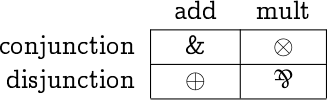Another way to classify the connectives is to say ⊕ and & are called additive, ⊗ and ⅋ are multiplicative, and ! and ? are called exponential.And still another classification says that ⊕, ⊗, and ! have positive polarity, while &, ⅋, and ? have negative polarity.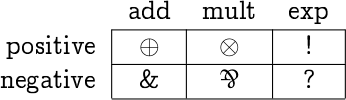This post will show that these arithmetical names are justified by analogy.

For one thing, multiplication-like connectives distribute over addition-like connectives.
2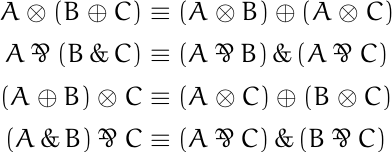Also, the exponential-like operators behave analogously to the equation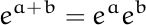with respect to the addition-like and multiplication-like connectives.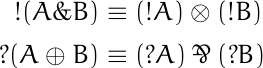In both equations, if you apply an exponential-like operator to the result of applying an addition-like operator, you get a multiplication-like operator applied to the exponential-like operator applied to two addition-like arguments separately.

The term “polarity” is justified by the fact that linear negation flips the polarity of connectives.

In the following analogs of De Morgan’s laws, negation turns conjunctions into disjunctions and vice versa, and it reverses the polarity of connectives.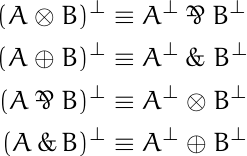Negating exponential connectives also flips polarity.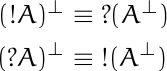These rules are analogous to the rules for negating quantifiers in classical logic.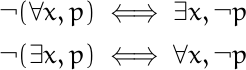# Names and numbers for modal logic axioms

Stanislaw Ulam once said

Using a term like nonlinear science is like referring to the bulk of zoology as the study of non-elephant animals.

There is only one way to be linear, but there are many ways to not be linear.

A similar observation applies to non-classical logic. There are many ways to not be classical.

## Modal logic axioms

Modal logic extends classical logic by adding modal operators □ and ◇. The interpretation of these operators depends on the application, but a common interpretation is “necessarily” and “possibly.”

There are a bewildering number of possible axioms to choose from in order to create a system of modal logic, and these often have cryptic names like “K”, “G”, and “5”. Seldom is any reason given for the names, and after digging into this a little, I think I know why: There isn’t much reason. Or rather, the reasons are historical rather than mnemonic.

For example, Axiom T is so named because Kurt Gödel published a paper that called some set of axioms “System T.” Of course that raises the question of why Gödel called his system “T.”

Axiom 5 gets its name from C. I. Lewis’ classification of logic systems. But Lewis’ numbering of systems S1 through S5 was essentially arbitrary, other than larger numbers corresponding to more axioms.

Unless you’re interested in the history for its own sake, it’s probably not worth questioning why rules are called what they are.

## Lemmon numbering

Although there’s not much logic behind the names of model logic axioms, there is a convenient way to catalog many of them. E. J. Lemmon  observed that many axioms for modal logic can be written in the formHere’s a list of some common modal logic axioms, along with their names and Lemmon numbers.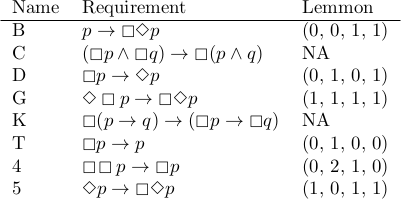E. J. Lemmon, An Introduction to Modal Logic, American Philosophical Quarterly, Monograph 11. Oxford, 1977.

# Why is the word problem hard?

This post is about the word problem. When mathematicians talk about “the word problem” we’re not talking about elementary math problems expressed in prose, such as “If Johnny has three apples, ….”

The word problem in algebra is to decide whether two strings of symbols are equivalent given a set of algebraic rules. I go into a longer description here.

I know that the word problem is hard, but I hadn’t given much thought to why it is hard. It has always seemed more like a warning sign than a topic to look into.

“Why don’t we just …?”

“That won’t work because … is equivalent to the word problem.”

In logic terminology, the word problem is undecidable. There can be no algorithm to solve the word problem in general, though there are algorithms for special cases.

So why is the word problem undecidable? As with most (all?) things that have been shown to be undecidable, the word problem is undecidable because the halting problem is undecidable. If there were an algorithm that could solve the word problem in general, you could use it to solve the halting problem. The halting problem is to write a program that can determine whether another arbitrary program will always terminate, and it can be shown that no such program can exist.

The impulse for writing this post came from stumbling across Keith Conrad’s expository article Why word problems are hard. His article goes into some of the algebraic background of the problem—free groups, (in)finitely generated groups, etc.—and gives some flavor for why the word problem is harder than it looks. The bottom line is that the word problem is hard because the halting problem is hard, but Conrad’s article gives you a little more of an idea what’s going on that just citing a logic theorem.

I still don’t have a cocktail-party answer for why the word problem is hard. Suppose a bright high school student who had heard of the word problem were at a cocktail party (drinking a Coke, of course) and asked why the word problem is hard. Suppose also that this student had not heard of the halting problem. Would the simplest explanation be to start by explaining the halting problem?

Suppose we change the setting a bit. You’re teaching a group theory course and the students know about groups and generators, but not about the halting problem, how might you give them a feel for why the word problem is hard? You might ask them to read Keith Conrad’s paper and point out that it shows that simpler problems than the word problem are harder than they seem at first.